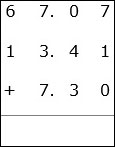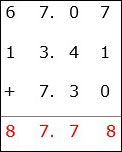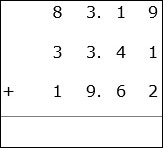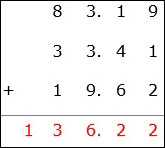# Decimal Addition With 3 Numbers

• The decimals are first aligned vertically so that digits of same place value are added.

• Zeros are added to the right of decimal numbers where ever necessary so that all numbers have the same number of digits after the decimal point.

Add 67.07 + 13.41 + 7.30

### Solution

Step 1:The decimals are aligned vertically above one another as shown.

Step 2:

We begin at the rightmost column 7 + 1 + 0 = 8; moving left 0 + 4 + 3 = 7; then

7 + 3 + 7 = 17. We bring down 7 and carry 1 to next column where 6 + 1 + 1 = 8.

Step 3:

We bring down 8 and get the final sum as shown.Add 83.19 + 33.41 + 19.62

### Solution

Step 1:The decimals are aligned vertically above one another as shown.

Step 2:

We begin at the rightmost column 9 + 1 + 2 = 12; We bring down 2 and carry 1 to next column; moving left 1 + 4 + 6 + 1 = 12; We bring down 2 and carry 1 to next column; next 3 + 3 + 9 + 1 = 16. We bring down 6 and carry 1 to next column where 8 + 3 + 1 + 1 = 13.

Step 3:

We bring down 13 and get the final sum as shown.